Hostname: page-component-cd4964975-8cclj Total loading time: 0 Render date: 2023-04-01T15:31:18.278Z Has data issue: true Feature Flags: { "useRatesEcommerce": false } hasContentIssue true

# Aperiodicity at the boundary of chaos

Published online by Cambridge University Press:  04 May 2017

## Abstract

We consider the dynamical properties of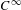$C^{\infty }$-variations of the flow on an aperiodic Kuperberg plug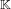$\mathbb{K}$. Our main result is that there exists a smooth one-parameter family of plugs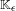$\mathbb{K}_{\unicode[STIX]{x1D716}}$ for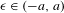$\unicode[STIX]{x1D716}\in (-a,a)$ and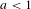$a<1$, such that: (1) the plug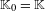$\mathbb{K}_{0}=\mathbb{K}$ is a generic Kuperberg plug; (2) for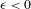$\unicode[STIX]{x1D716}<0$, the flow in the plug$\mathbb{K}_{\unicode[STIX]{x1D716}}$ has two periodic orbits that bound an invariant cylinder, all other orbits of the flow are wandering, and the flow has topological entropy zero; (3) for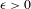$\unicode[STIX]{x1D716}>0$, the flow in the plug$\mathbb{K}_{\unicode[STIX]{x1D716}}$ has positive topological entropy, and an abundance of periodic orbits.

Type
Original Article
Information
Ergodic Theory and Dynamical Systems , October 2018 , pp. 2683 - 2728

## Access options

Get access to the full version of this content by using one of the access options below. (Log in options will check for institutional or personal access. Content may require purchase if you do not have access.)

## References

Bowen, R.. Entropy for group endomorphisms and homogeneous spaces. Trans. Amer. Math. Soc. 153 (1971), 401414.CrossRefGoogle Scholar
Edwards, R., Millett, K. and Sullivan, D.. Foliations with all leaves compact. Topology 16 (1977), 1332.CrossRefGoogle Scholar
Epstein, D. B. A. and Vogt, E.. A counterexample to the periodic orbit conjecture in codimension 3. Ann. of Math. (2) 108 (1978), 539552.CrossRefGoogle Scholar
Ghys, É.. Construction de champs de vecteurs sans orbite périodique (d’après Krystyna Kuperberg), Séminaire Bourbaki, Vol. 1993/94, Exp. No. 785. Astérisque 227 (1995), 283307.Google Scholar
Hurder, S. and Rechtman, A.. The dynamics of generic Kuperberg flows. Astérisque 377 (2016), 1250.Google Scholar
Hurder, S. and Rechtman, A.. Perspectives on Kuperberg flows. Preprint, 2016, arXiv:1607.00731, submitted.Google Scholar
Katok, A.. Lyapunov exponents, entropy and periodic orbits for diffeomorphisms. Publ. Math. Inst. Hautes Études Sci. 51 (1980), 137173.CrossRefGoogle Scholar
Kuperberg, K.. A smooth counterexample to the Seifert conjecture. Ann. of Math. (2) 140 (1994), 723732.CrossRefGoogle Scholar
Kuperberg, G. and Kuperberg, K.. Generalized counterexamples to the Seifert conjecture. Ann. of Math. (2) 144 (1996), 239268.CrossRefGoogle Scholar
Matsumoto, S.. K. M. Kuperberg’s C counterexample to the Seifert conjecture. Sūgaku, Math. Soc. Japan 47 (1995), 3845; Translation: Sugaku expositions, Amer. Math. Soc. 11, (1998) 39–49.Google Scholar
Matsumoto, S.. The unique ergodicity of equicontinuous laminations. Hokkaido Math. J. 39 (2010), 389403.CrossRefGoogle Scholar
Sullivan, D.. A counterexample to the periodic orbit conjecture. Publ. Math. Inst. Hautes Études Sci. 46 (1976), 514.CrossRefGoogle Scholar
Walters, P.. An Introduction to Ergodic Theory (Graduate Texts in Mathematics, 79) . Springer, New York, 1982.CrossRefGoogle Scholar
Wilson, F. W. Jr. On the minimal sets of non-singular vector fields. Ann. of Math. (2) 84 (1966), 529536.CrossRefGoogle Scholar